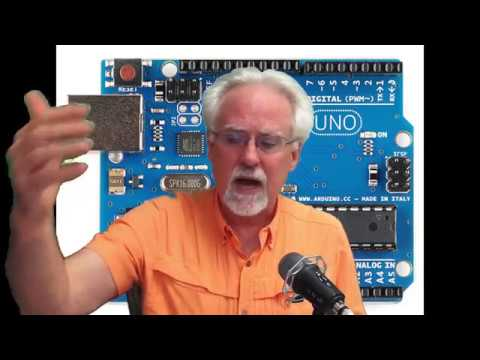# Arduino Tutorial 7: Understanding the Arduino Analog Write CommandIn earlier lessons we learned how to turn pins on and off with the Arduino digitalWrite command. This is great if you only want 0 or 5 volts. In this lesson we show how you can get the in between values by using the analogWrite command. In later lessons we will show more specifically how analogWrite works, but this present lesson gets you started, and shows you how to control brightness of an LED. In all these lessons we are using the Arduino Super Starter Kit, which you can pick up HERE.